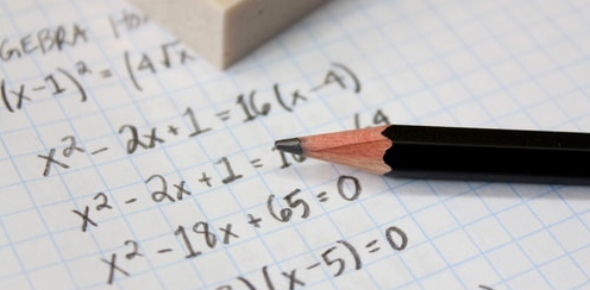# Math Exam Practice Quiz!

15 Questions | Total Attempts: 103Settings.

• 1.
Find the remainder when 1075 + 875 is divided by 9.
• A.

0

• B.

1

• C.

2

• D.

None

• 2.
Find the number of two-digit and three-digit factors of 200.
• A.

12

• B.

10

• C.

8

• D.

7

• 3.
After the division of a number successively by 2, 5 and 4 the remainders obtained are 1, 3 and 2 respectively. What will be the remainderswhen the same number is divided by 5, 2 and 4.
• A.

2, 1, 2

• B.

2, 0, 1

• C.

1, 2, 0

• D.

None

• 4.
A certain number is divided by 111 leaves the remainder 59. Find the remainder when the same number is divided by 37?
• A.

22

• B.

59

• C.

50

• D.

36

• 5.
Find the unit digit of (299 * 5 99 * 357 * 666 )
• 6.
What is the remainder when 3388 X 2883 X 1817 is divided by 11?
• A.

1

• B.

3

• C.

2

• D.

None

• 7.
The sum of digits of a number N is 70254. Which of the following is/are definitely true about the number N?
• A.

Abcd is a multiple of 9

• B.

Abcd is a multiple of 3

• C.

Abcd is a multiple of 11

• D.

Abcd is a prime number

• 8.
Find the remainder when 10785 + 8716 is divided by 9.
• A.

0

• B.

1

• C.

2

• D.

None

• 9.
Find the unit digit of 123456789(odd*Even)
• 10.
Find the highest power of 30 in 10!
• A.

3

• B.

2

• C.

4

• D.

None

• 11.
A number when divided successively by 4 and  5 leaves remainders 1 and 4 respectively. When it is successively divided by 5 and 4, then the respective remainders will be
• A.

1, 2

• B.

2, 3

• C.

3, 2

• D.

4, 1

• 12.
On dividing a number by 5, we get 3 as remainder. What will the remainder when the square of this number is divided by 5 ?
• A.

5

• B.

0

• C.

4

• D.

1

• 13.
Let N be the greatest number that will divide  1305, 4665 and 6905, leaving the same remainder in each case. Then sum of the digits in N is:
• A.

4

• B.

5

• C.

6

• D.

8

• 14.
A, B and C start at the same time in the same direction to run around a circular stadium. A completes a round in 252 seconds, B in 308 seconds and c in 198 seconds, all starting at the same point. After what time will they again at the starting point ?
• A.

26 minutes and 18 seconds

• B.

42 minutes and 36 seconds

• C.

45 minutes

• D.

46 minutes and 12 seconds

• 15.
The product of two numbers is 2028 and their H.C.F. is 13. The number of such pairs is:
• A.

1

• B.

2

• C.

3

• D.

4

Related TopicsBack to top